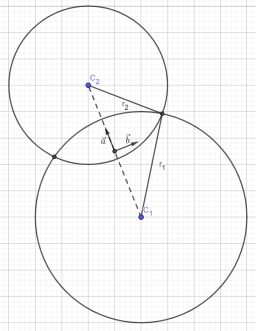# Find all 2

Find all the complex solutions (write the answer in the form x+iy) of the system.
{|z-12|/|z-8i|=5/3 ; |z-4|=|z-8|

z1 = 6 + 8 i
z2 = 6 + 17 i

### Step-by-step explanation:Did you find an error or inaccuracy? Feel free to write us. Thank you!

Tips for related online calculators
Are you looking for help with calculating roots of a quadratic equation?
Do you have a linear equation or system of equations and looking for its solution? Or do you have a quadratic equation?
Try our complex numbers calculator.
The Pythagorean theorem is the base for the right triangle calculator.

#### Grade of the word problem:

We encourage you to watch this tutorial video on this math problem: